Add Data in Excel  Step by Step Guide ▷ 2020

This program of Microsoft Office has become the right hand of most of people and especially for large companies who need to make a big number of calculations. And it is that this software stands out mainly for offering many tools that will allow you to perform mathematical operations very easily.

When working with numerical data in software on many occasions they have to do some sums, this is a very simple process to do which will allow you automate your accounts and above all it will offer you greater speed when working on the spreadsheet.

However, not all users know how to add in excel, so it turns out very important to explain this process. That is why here we are going to teach you step by step how you can make additions in the program and what are the formulas you can use for it.

What are the main Excel formulas for summing data in spreadsheets?

One of the main advantages that can be obtained when working with this microsoft program, is that it offers a great variety of functions and formulas that will allow different types of operations math and calculations in a very simple way.

In the case of sums can be applied for many methods, that is why here we are going to teach you what are the main formulas you can find there so you can use in each of your worksheets.

Let’s see below:

SUM function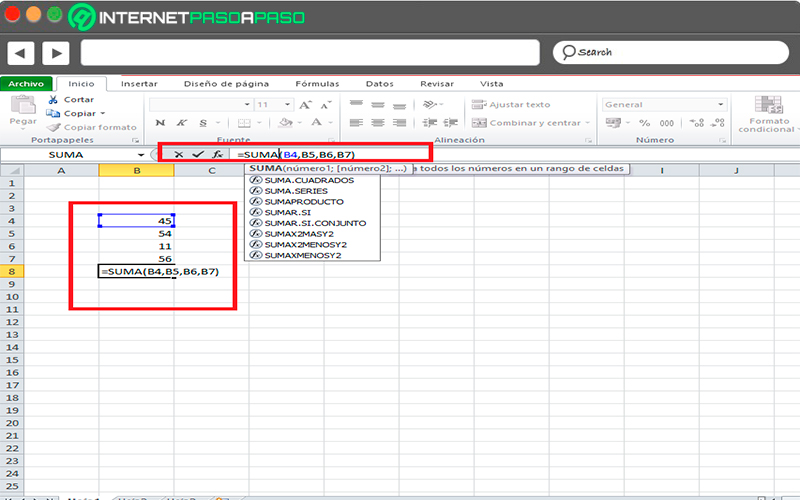This formula is used for those cases where you want add two or more cells in your worksheet. To be able to apply it you must write it in the following way: = SUM (numbers Y letters of the cells to be added separated by a comma) this would be as follows: = SUM (B4, B5, B6, B7)

In this way it will be possible to add all the values ​​of the cells that have been introduced in the equation.

Use the + sign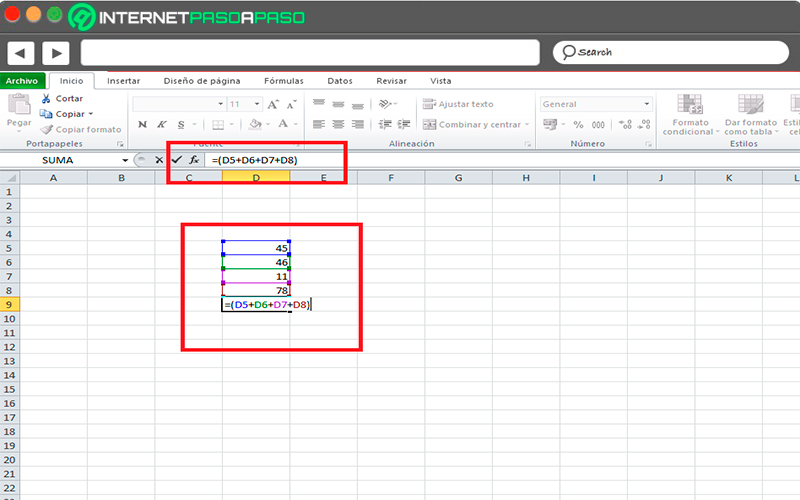Another way you can add values ​​contained in spreadsheets it is through plus sign, for this you must click on the cell where do you want me show you the result, the next thing will be to go to the option of “Insert function”.

There you must write the sign = and select each of the boxes that you want to add, once selected the first one you must press the key with the + sign so that it separates each of the values.

When all the corresponding values ​​have been entered, simply press the “Enter” key this way you can see how the result of the sum of all the values ​​is reflected that you have previously selected.

SUMIF function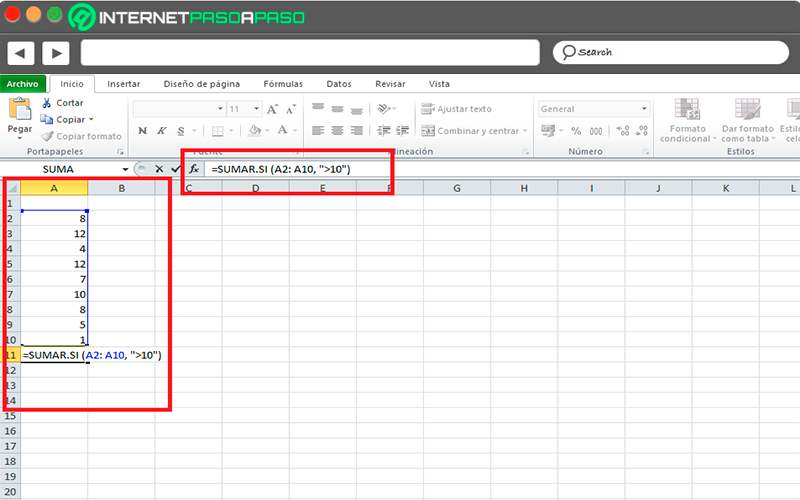Another function that can be used to add values ​​is ADD IF, it allows sum values ​​of an interval what they meet previously specified criteria.

An example of this would be that, in one of the columns from the spreadsheet has only numbers and you need only add the values ​​that are greater than 10, for this you would have to apply the following formula: = ADDIF (A2: A10, “> 10”)

This is another of the mathematical functions that you can add in each of your criteria at the time of work with excel. In this case you can use this function to add the number of retailers in the country that have a single postal code, and the profits that exceed a certain amount in euros.

The formula in this case would be the following: ADD.IF.SET (sum_range, criteria_range1, criteria1, [rango_criterios2; criterios2]; ….)

SUM.SERIES

This is another formula available to carry out sum in Excel.

In this case it is the sum of power that has the following formula:

• SUM.SERIES (x, n, m, coefficient)

Each of these values ​​has the following argument:

• X: Mandatory, is the variable used as base on the exponential series.
• N: Mandatory, is the initial exponent to which base x is raised.
• M: Required, is the step in which increases the value of n for each term in the series.
• Coefficient: Required, this will be the coefficient by which each of the successive exponents of X. the number of values ​​in the argument would be exponential.

Fortunately, Excel offers the possibility of add up virtually any data found on your spreadsheet. That is why here we are going to teach you several methods that you can perform to add data in the program, in this case it is add figures, dates, hours, among other data that you can also add.

To do this, follow each of these methods that we will teach you below:

Figures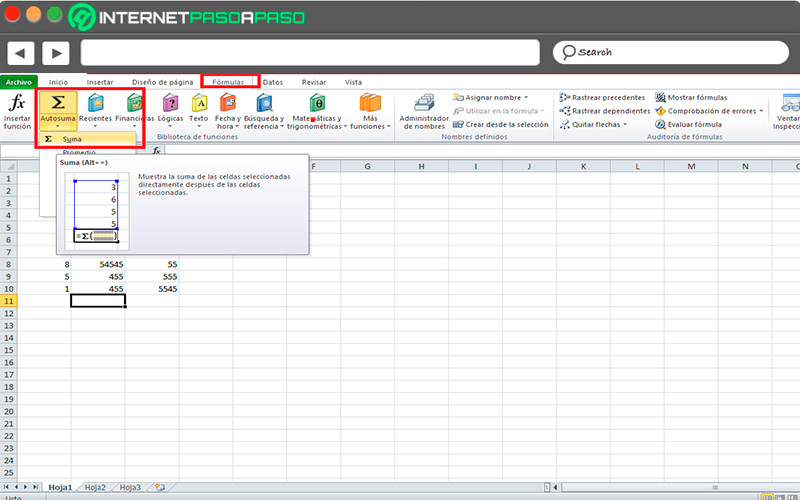One of the ways faster and easier to add figures in the calculation software is through the tool “Autosum”, for this you must select a cell empty that is below the column of data.

The next thing will be to go to the tab “Formulas” and there you select the option of “Autosum” and then “Sum”.

In this way Excel will automatically detect the interval to be added. It also can work horizontally, for this you must select a empty cell to the right of the row.

Dates

The dates are other elements that can be add in excel, in this case this procedure is performed with the sum of the values ​​of a certain date or the sum of the values ​​within a previously selected date range.

In this case it is necessary to make use of the SUMIF function, which allows set the sum under a specific condition.

An example of all this would be the following:

• Having the data what are we going to teach you next the sum of all sales made before 04/01/2015 will be drawn and I also know will add all sales made on 05/01/2015.
• In order to determine the sum of sales before the day 01/04/2015, it is necessary to use this function: = ADDIF (A2: A12; “
• Now in order to obtain the sum of all sales made on the day 01/05/2015 it will be necessary to write the following function: = ADDIF (A2: A12; “=” & DATE (2015; 1; 5); B2: B12).

You can too handle excel dates as a number and in it use the addition and subtraction operations.

In the case of doing sums, you will need to follow these steps:

• Write the date in cell A2, either 05/19/2019.
• The next thing will be to click on it with the right mouse button and select the option “Cell format”.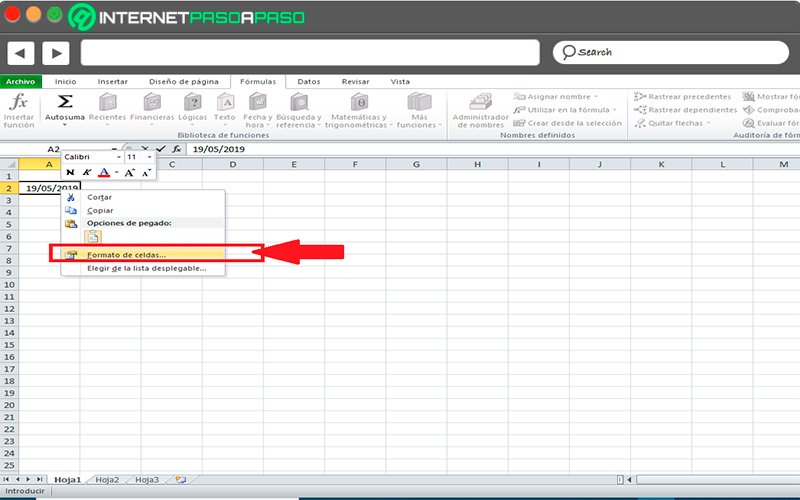• Now select within the tab the number of a short date format. You can too to select said option in the number option group drop down menu, there you choose “Short date”.
• Now select cell B1 and write the following operation = A2 + 2, as you can see automatically se add the days to the date what do you have in the column A.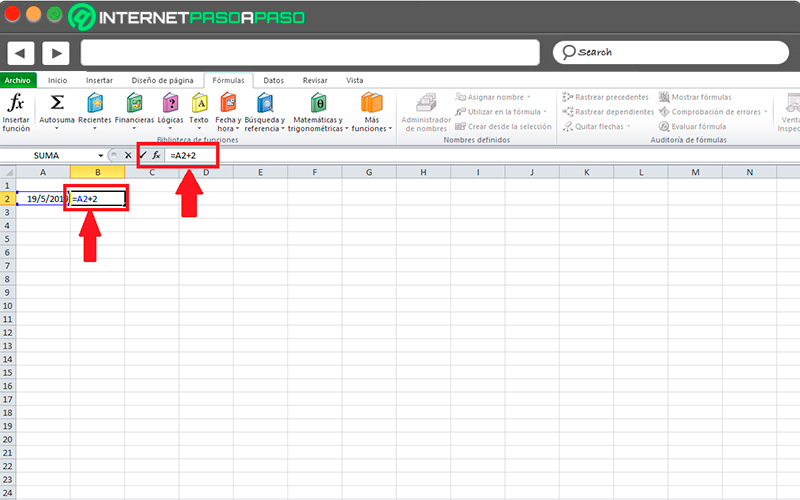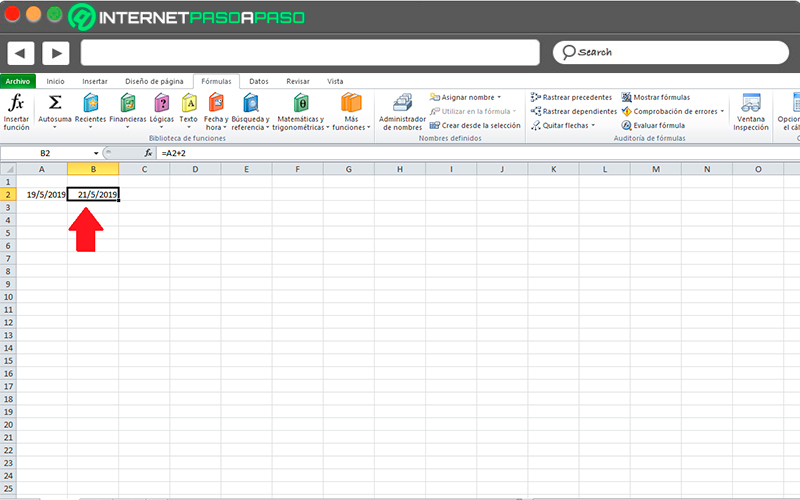Hours

Power add hours in Microsoft Excel It is not such a simple task, since for this it will be necessary apply some tricks that will allow you to do it the form correct. In this case it is recommended first add the minutes and then convert it to hours.

Although it should be mentioned that the biggest problem here occurs when the sum must be done with several cells containing hours and minutes. Note that every 60 minutes will be one hour, this ends up complicating what will be the sum of these values.

Therefore, if you have a cell range with hours and minutes in which a person has worked for a week in the company as you can see in the picture.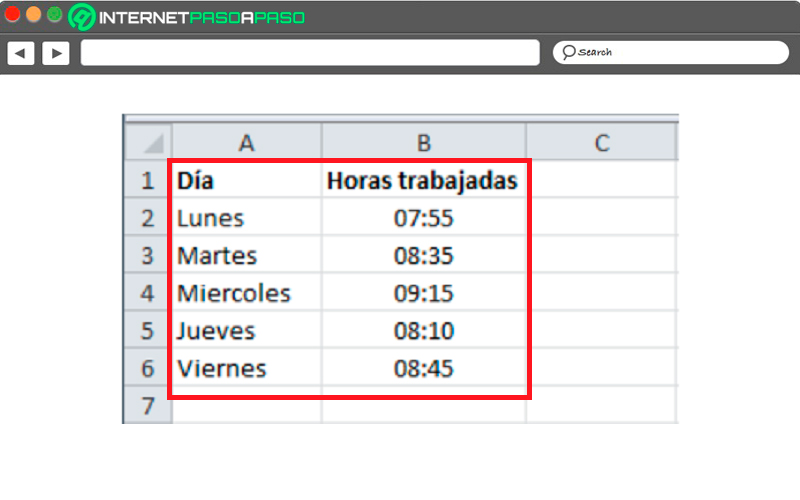So for the boss can make the payment of the fees it will be necessary to be clear the correct sum of the time you have worked. Here we are going to explain the easiest method to add the hours and minutes.

In the cell B7 you must use the SUM function, this will allow add up all the values. Here you can see how the cell shows a time that does not correspond to the correct sum.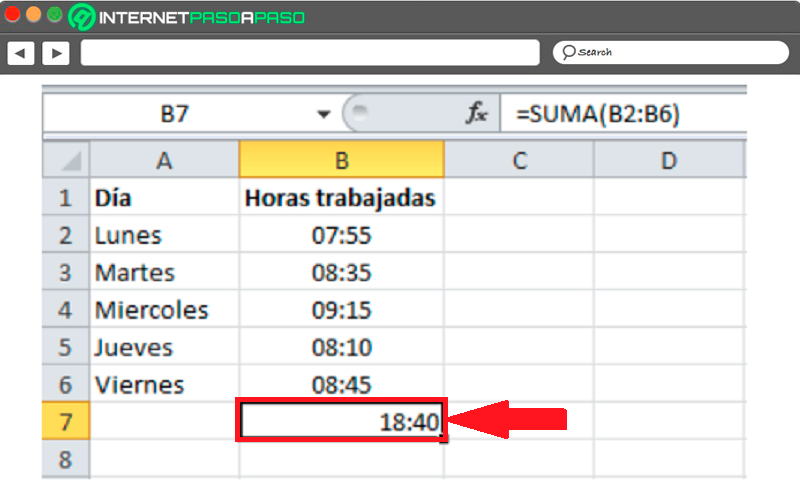For this value of correctly you need to right click on cell B7, there choose the option of “Cell format” and select the section of “Number” and then the option of “Personalized” so you can finally choose the format [H]: mm; ss.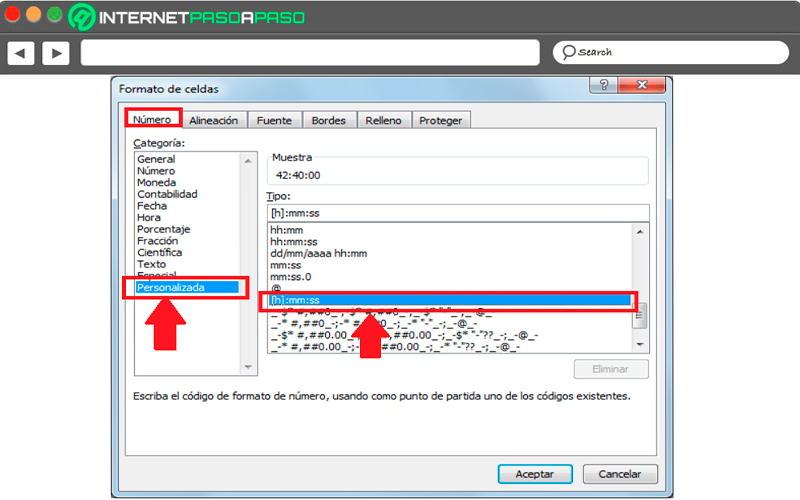In the event that for some reason don’t get the format, what you should do is capture it in the text box. As you can see, this format places the symbol for hours in square brackets, which allows to show the correct result of the sum, when finished click on “To accept” for the result to be generated.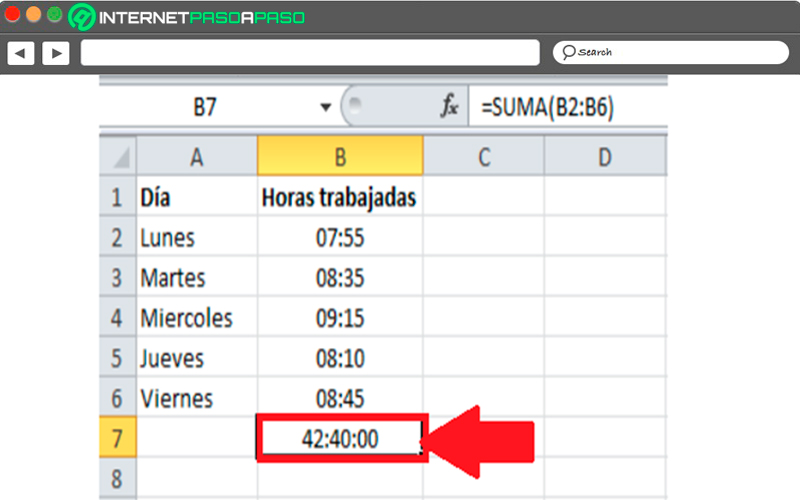In this way you can start to make las sums of hours and minutes in Excel without the need to carry out very complex procedures. As you can see, just enough modify the format of the cell that will show the final sum of the hours and minutes.

In case you want add minutes and hours you can apply the same trick but selected the correct format for it.

If you have any questions, leave them in the comments, we will answer you as soon as possible, and it will surely be of great help to more members of the community. Thank you! 😉

Rate this post